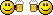###Author Topic: Is Number Entered a whole number or not?  (Read 6769 times)

####mrplant##### Is Number Entered a whole number or not?
« on: 2013-Aug-13 »
My brains gone to sleep today.
User enters a number at the keyboard. Its  a real number. Say 10.2 or 10.6 or 10.8 or 11.4 - How do I reject any input that isn't a whole exact number?
I am trying to use FMOD but my formula must be dodgy..

Anyone?
« Last Edit: 2013-Aug-13 by mrplant »

####erico

• Community Developer
• Prof. Inline
••• Posts: 4396##### Re: Is Number Entered a whole number or not?
« Reply #1 on: 2013-Aug-13 »
With the INTEGER() command.
Do you need to round it to the closest integer or just get rid of the decimals?

edit: oh wait...do you mean not to accept the number at all if it is not an integer?

####mrplant##### Re: Is Number Entered a whole number or not?
« Reply #2 on: 2013-Aug-13 »
I am writing a calculator for doing the change machine at work..

What I am doing is asking the user how many 20p coins they have.
They enter say 6
This makes 0.20 x 6 = 1.20
I want to reject that input and get them to re-enter it. (The machine should always hold an exact not a fractional part of a pound in coins)
valid inputs would be a number of twenty pence coins that come to an exact pound.
A total amount of coins that comes to 1.20 or 1.40 or 1.60 or 1.8 are all invalid.

I know the solution is integer related but my brain has failed me today..

This isn't working properly:

IF INTEGER((TwentyPences*0.20)-INTEGER(TwentyPences*0.20)) <> 0 // If  we have a non exact amount here eg 79.80 instead of 80.00 pounds for example.
// Print "(STRANGE TOTAL - PLEASE CHECK MACHINE AGAIN"

Well its working perfectly in fact - just not giving the the results I need lol!
« Last Edit: 2013-Aug-13 by mrplant »

####erico

• Community Developer
• Prof. Inline
••• Posts: 4396##### Re: Is Number Entered a whole number or not?
« Reply #3 on: 2013-Aug-13 »
hmm, that example should work I guess?
But I had my share on integer alien outcomes and am not that good in math at all.

Let´s say, don´t calculate inside the integer command brackets. Can you give that exact idea a try but with variables outside?

####mrplant##### Re: Is Number Entered a whole number or not?
« Reply #4 on: 2013-Aug-13 »
Silly me - I was over thinking the problem..

Since I took input in 20pences and 5 make a pound, I just had to look at the modulus after dividing by 5 for my answer...

IF MOD(TwentyPences,5) <> 0 // If remainder of dividing by 5 is not zero, we have a non exact amount here eg 79.80 instead of 80.00 pounds for example.

####erico

• Community Developer
• Prof. Inline
••• Posts: 4396##### Re: Is Number Entered a whole number or not?
« Reply #5 on: 2013-Aug-13 »
Good it is auto solved!...And I also had a chance to learn some more math, didn´t know about MOD.####Moru

• Prof. Inline
••• Posts: 1791##### Re: Is Number Entered a whole number or not?
« Reply #6 on: 2013-Aug-14 »
Oh, I wrote something like this for a company a few years ago. Luckily in Sweden we don't have many different coins, makes the change-calculation much easier :-)

I had one input-box for each coin and note type.
0.5 = [empty box]
1 = [empty box]
5 = [empty box]
10 = [empty box]
20 = [empty box]
50 = [empty box]
100 = [empty box]
500 = [empty box]

The input box only accepted numbers, nothing else so when user tried to type . or , there was no output in the box.
Make it easy for the user, avoid error messages if you can. Prevents the error from occuring at all.

Of course this was written in some old VisualBasic version at that time. Don't think GLBasic even existed then :-)

####Moru

• Prof. Inline
••• Posts: 1791##### Re: Is Number Entered a whole number or not?
« Reply #7 on: 2013-Aug-14 »
I was thinking something like this:

Code: (glbasic) [Select]
`GLOBAL number\$, in\$, asciiWHILE TRUE in\$=INKEY\$() // get keypress IF in\$<>"" ascii = ASC(in\$) // convert to ascii DEBUG ASC(in\$) + "\n" // debug print just to see all ascii codes for all keys you press IF ascii=8 // Backspace is 8 number\$=MID\$(number\$, 0, LEN(number\$)-1) // remove last character ENDIF IF ascii>=ASC("0") AND ascii<=ASC("9") // We have a number so add it to the string number\$ = number\$ + in\$ ENDIF ENDIF PRINT "Number: " + number\$, 0, 100 SHOWSCREENWENDEND`

####Slydog

• Prof. Inline
••• Posts: 932
• KodeSource##### Re: Is Number Entered a whole number or not?
« Reply #8 on: 2013-Aug-14 »
Another solution would be:

Code: (glbasic) [Select]
`numberOfCoins# = 6 // Or whatever number the user enteredamount# =  numberOfCoins# * 0.20 // The total amount in 'p', which is 6 * 0.2, or 1.2 in this caseIF INTEGER(amount) = amount THEN   // Valid value enteredENDIF`
So, with the above numbers, INTEGER(1.2) = 1.2 is FALSE since INTEGER(1.2) = 1.
But if numberOfCoins is say 10 then INTEGER(2.0) = 2.0 is TRUE since INTEGER(2.0) = 2.

Of course the modulus operator would work also, as you found out.
In the above code, I could have also said: (FMOD works with floating point numbers)
IF FMOD(amount, 1.0) = 0 THEN ... // We have a full dollar/pound/Euro amount
This checks to see if any pennies (or pence?) are remaining from a value.
My current project (WIP) :: TwistedMaze <<  [Updated: 2015-11-25]

####mrplant##### Re: Is Number Entered a whole number or not?
« Reply #9 on: 2013-Aug-15 »
Interesting answers here - thanks .